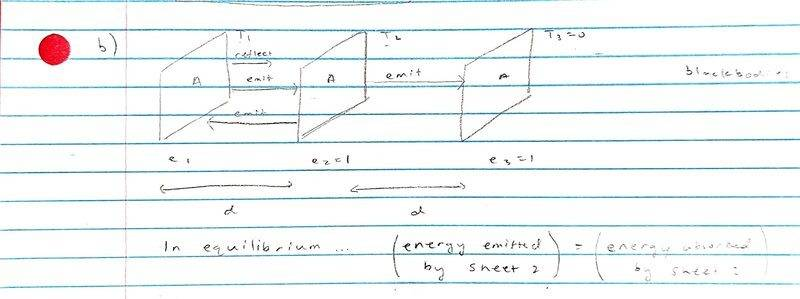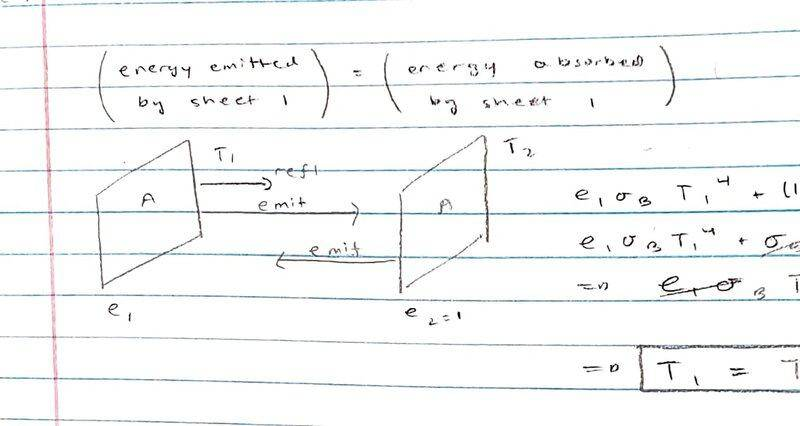# 0 power emitted between 3 blackbody sheets (Stefan-Boltzmann radiation)

baseballfan_ny
Homework Statement:
A sheet (“sheet 1”) of area A having emissivity ##e_1## is held at temperature ##T_1## so that it emits a total power ##P_1## to the right (i.e., energy per unit time). Let σB be the Stefan-Boltzmann constant and neglect all edge effects.

a. In terms of ##P_1##, calculate temperature ##T_1## of sheet 1.

Two additional black sheets, each of area A and having emissivities ##e_2## = 1 and ##e_3## = 1, are now added to the right of sheet 1. (See figure below) The spacings between the pairs of sheets is d, such that ##d^2## is much less than A . The temperature ##T_2## of the middle sheet (2) is allowed to vary and the right-hand sheet (3) is held at a fixed temperature ##T_3## = 0.

b. Assume that sheet 2 reaches a steady state temperature ##T_2##. What is the power flow ##P_{23}## (total power, not power per unit area) emitted by sheet 2 to the right-hand sheet, i.e., from sheet 2 to sheet 3? Your answer should be given in terms of in terms of ##T_2##, A, d, ##\sigma_B##, and ##e_1## only. [Note: Not all of these parameters should appear in your answer.]

c. Determine ##T_2## in terms of ##T_1##, A, d, ##\sigma_B## and ##e_1## only.
Relevant Equations:
Stefan-Boltzmann
## J_u = e \sigma_B T^4 ##
I need someone to check my work, because I'm getting weird results that I'm not able to interpret physically for parts b and c. Thanks in advance.

For part a...

##J_u = e_1 \sigma_B T^4##
##P_1 = AJ_u = e_1 \sigma_B AT_1^4##
## T_1 = \left( \frac {P_1} {e_1 \sigma_B A} \right)^{\frac 1 4} ##

For part b...I'm using the idea that energy emitted by sheet 2 = energy absorbed by sheet 2

## \sigma_B T_2^4 + \frac {P_{23}} {A} = e_1 \sigma_B T_1^4 + (1 - e_1) \sigma_B T_2^4 ##
## \frac {P_{23}} {A} = e_1 \sigma_B \left( T_1^4 - T_2^4 \right) ##
## P_{23} = A e_1 \sigma_B \left( T_1^4 - T_2^4 \right) ##

For part c...I'm using the idea that energy emitted by sheet 1 = energy absorbed by sheet 1

## e_1 \sigma_B T_1^4 + \left( 1 - e_1 \right) \sigma_B T_2^4 = \sigma_B T_2^4 ##
## e_1 \sigma_B T_1^4 = e_1 \sigma_B T_2^4 ##
## T_1 = T_2##

Now this has been bothering me, because if ##T_1 = T_2##, then ##P_{23} = 0##? I can't seem to make sense of that.

Homework Helper
Gold Member
2022 Award
For part b...
View attachment 291135
I'm using the idea that energy emitted by sheet 2 = energy absorbed by sheet 2

## \sigma_B T_2^4 + \frac {P_{23}} {A} = e_1 \sigma_B T_1^4 + (1 - e_1) \sigma_B T_2^4 ##
I don't see how you get this equation. Surely emissions from the third sheet are part of the middle sheet's energy balance. And can't you immediately write down how ##P_{23}## depends on ##T_2## without reference to the other sheets?

baseballfan_ny
Surely emissions from the third sheet are part of the middle sheet's energy balance.
But ##T_3## = 0, so it doesn't emit any energy, right? I suppose it can absorb energy emitted by ##P_{23}##.

And can't you immediately write down how P23 depends on T2 without reference to the other sheets?
I had thought that originally ... that ##P_{23} = A\sigma_B T_2^4##, but I think there has to be equilibrium maintained? So that's why applied those conditions.

I just don't think it makes sense for ##P_{23} = 0##.

Homework Helper
Gold Member
2022 Award
But T3 = 0
Sorry, I missed that.
I had thought that originally ... that ##P_{23} = A\sigma_B T_2^4##, but I think there has to be equilibrium maintained?
That equation is a valid answer whether or not equilibrium is maintained. The answer you gave includes T1, which is not allowed.

I'm using the idea that energy emitted by sheet 1 = energy absorbed by sheet 1

## e_1 \sigma_B T_1^4 + \left( 1 - e_1 \right) \sigma_B T_2^4 = \sigma_B T_2^4 ##
Please explain how you get that equation.
One thing I am not clear on is whether you are supposed to assume all arriving energy is absorbed, or whether in each case absorptivity equals emissivity, so (1-e) would be reflected (much complicating matters).

baseballfan_ny
Please explain how you get that equation.
My idea was that the total amount of energy emitted by sheet 1 = total amount of energy "delivered" to sheet 1 (I realized I wrote "absorbed" instead of "delivered" in Post 1 so that was probably confusing). The first term is the energy emitted by sheet 1, since it has absorptivity = emissivity = ##e_1##. The 2nd term was the amount of energy (originating from sheet 2) reflected by sheet 1. The right hand side is the amount of energy delivered to sheet 1 from sheet 2.
One thing I am not clear on is whether you are supposed to assume all arriving energy is absorbed, or whether in each case absorptivity equals emissivity, so (1-e) would be reflected (much complicating matters).
I believe I'm supposed to take ##a = e## by Kirchoff's Law.

That equation is a valid answer whether or not equilibrium is maintained. The answer you gave includes T1, which is not allowed.
Ok so I'm getting something new. I think I'm supposed to say sheet 2 emits ##J = \sigma_B T_2^4## and then enforce equilibrium.

So then my answer to part b would just be...
## P_{23} = A \sigma_B T_2^4 ##.

Then for part c I would enforce equilibrium on sheet 2... that however much is absorbed by it is emitted...

##(1 - e_1)\sigma_B T_2^4 + e_1 \sigma_B T_1^4 = \sigma_B T_2^4 ##

where the first term is the amount reflected by sheet 1 and reabsorbed by sheet 2, the second term is the amount emitted by sheet 1 and absorbed by sheet 2, and the right hand side term is the amount emitted by sheet 2.

So...

## \sigma_B T_2^4 - e_1\sigma_B T_2^4 + e_1\sigma_B T_1^4 = \sigma_B T_2^4 ##

##- e_1\sigma_B T_2^4 + e_1\sigma_B T_1^4 = 0 ##

##T_1 = T_2##, but as you pointed out, ##P_{23} = A \sigma_B T_2^4## and should not be in terms of ##T_1## so I no longer have ##P_{23} = 0##